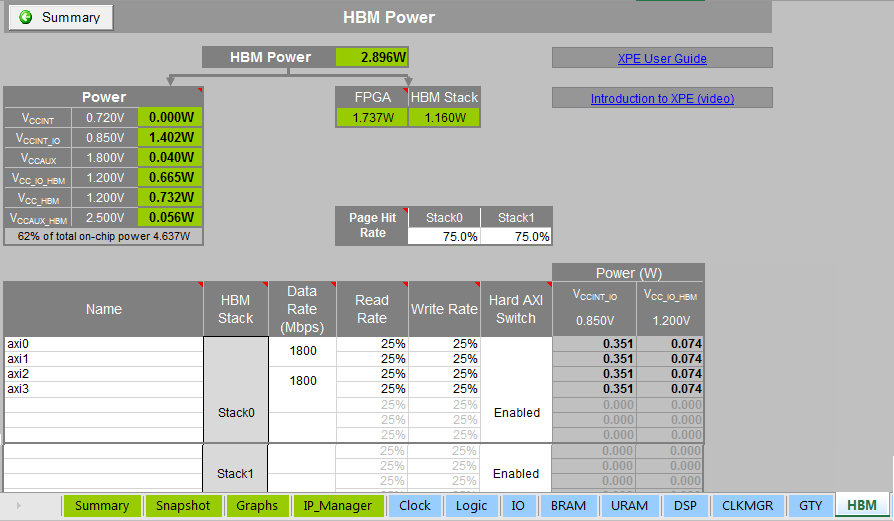# Estimating HBM Power (HBM Sheet) - 2022.1 English

## Xilinx Power Estimator User Guide (UG440)

Document ID
UG440
Release Date
2022-04-26
Version
2022.1 English

The HBM sheet is available only for Virtex UltraScale+ HBM devices. This sheet is used to estimate the power of High Bandwidth Memory. Each HBM device contains one or two 32 Gb memory stacks. The following figure shows the HBM sheet for a VU37P device which contains two memory stacks. To enter HBM IP information in XPE, use the Add HBM Wizard. The Add HBM Wizard allows you to configure HBM design to a granular level. For more information on how to use the Add HBM Wizard, see Using the Add HBM Wizard.

Figure 1. HBM SheetThe HBM Power sheet displays the total dynamic power of the HBM subsystem. The total dynamic power can be further partitioned to show HBM stack power and the FPGA portion which is from the dedicated interface logic. The Power table shows the dynamic power dissipated on each power rail.

The Page Hit Rate is the estimated rate of a memory transaction to access an open page,which results in the fastest access. For example, sequential memory accesses are more likely to occur within an open page which reduces power and increases efficiency. The default value is 75% for each stack.

In the main table, each row represents a pseudo-channel associated with an AXI port capable of accessing a contiguous 2Gb section of an HBM stack. There are 16 pseudo-channels associated with Stack0 and 16 associated with Stack1. Each of the 16 psuedo-channels must access the HBM using one of the eight dedicated memory controllers and each memory controller simultaneously accesses two HBM 2Gb sections.

Data Rate for each memory controller is specified in Mb/s. The valid range is from 100 to 1800 (1600 for -1 device speed). There can be different rates within the same stack. However, they must be integer multiples (if the rates are different). For example, if a stack has a memory controller with a rate of 1800, the next slower valid rate is 900 (1/2) and the next slower rate after that is 450 (1/4) and so on.

The default value of Read Rate and Write Rate are set to 25%. However, the maximum rate also depends on the page hit rate. The valid condition for Read and Write rates are as follows:

• If the Page Hit Rate is less than 75%, the (Read Rate + Write Rate) is less than or equal to 50%

• If the Page Hit Rate is greater than or equal to 75%, the (Read Rate + Write Rate) is less than or equal to 90%

If the above conditions are not met, the Read Rate and Write Rate cells are marked with a dark yellow warning color. However, the power numbers displayed are still valid.